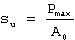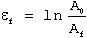# True Stress – True Strain Curve: Part Three

The parameters that are usually determined from the true stress – true strain curve include true stress at maximum load, true fracture stress, true fracture strain, true uniform strain, true local necking strain, strain-hardening exponent and strength coefficient.

### True Stress at Maximum Load

The true stress at maximum load corresponds to the true tensile strength. For most materials necking begins at maximum load at a value of strain where the true stress equals the slope of the flow curve. Let su and eu denote the true stress and true strain at maximum load when the cross-sectional area of the specimen is Au. The ultimate tensile strength is given byEliminating Pmax yields

(1)True Fracture Stress

The true fracture stress is the load at fracture divided by the cross-sectional area at fracture. This stress should be corrected for the, triaxial state of stress existing in the tensile specimen at fracture. Since the data required for this correction are often not available, true-fracture-stress values are frequently in error.

True Fracture Strain

The true fracture strain ef is the true strain based on the original area A0 and the area after fracture Af

(2)This parameter represents the maximum true strain that the material can withstand before fracture and is analogous to the total strain to fracture of the engineering stress-strain curve. Since Eq. (3) is not valid beyond the onset of necking, it is not possible to calculate ef from measured values of ef. However, for cylindrical tensile specimens the reduction of area q is related to the true fracture strain by the relationship

(3)True Uniform Strain

The true uniform strain eu is the true strain based only on the strain up to maximum load. It may be calculated from either the specimen cross-sectional area Au or the gage length Lu at maximum load.

Equation (3) may be used to convert conventional uniform strain to true uniform strain. The uniform strain is often useful in estimating the formability of metals from the results of a tension test.

(3)True Local Necking Strain

The local necking strain en is the strain required to deform the specimen from maximum load to fracture.

(5)